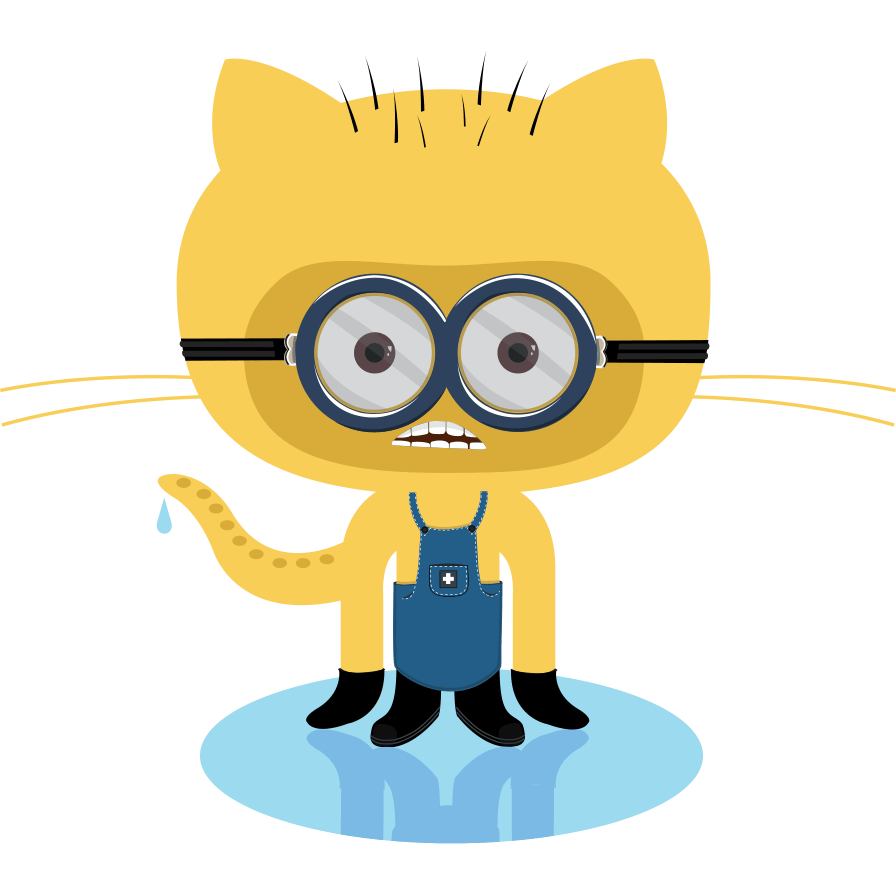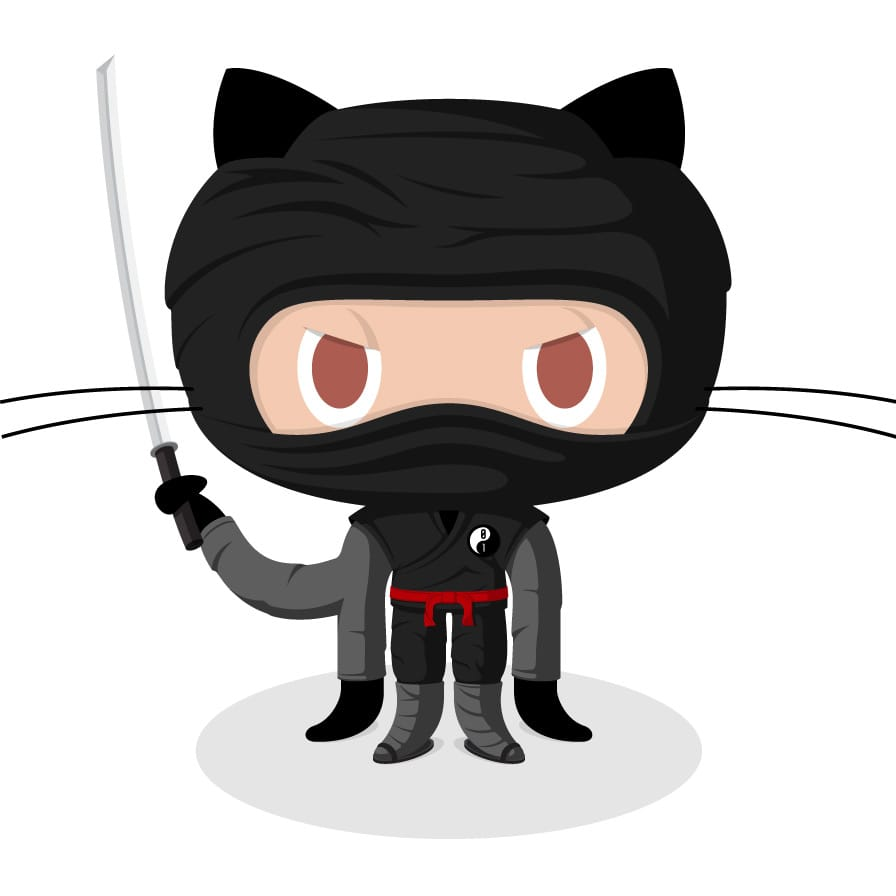28 Aug 2016 by Louis

## Introduction

This is a style guide to help in formatting Markdown content for the site. This page is very similar to the style cheatsheet on Trevor Bedford’s site. In general, I’ve tried to adhere to GitHub style, with the intention that anything that renders on GitHub will render here as well. The following isn’t meant to be exhaustive, just a reminder of what’s possible with Markdown.

# Heading 1


## Text styling

Italicize text by surrounding it in *asterisks* or _underscores_.
Bold text by surrounding it with **double asterisks** or __double underscores__.
Combine with **_double asterisks plus underscores_**.
Strike-through text with ~~double tildes~~.
Superscript contiguous text with carrots like x^2y, surround with parentheses for more complex expressions like x^(2y + 3z).


Italicize text by surrounding it in asterisks or underscores.

Bold text by surrounding it with double asterisks or double underscores.

Combine with double asterisks plus underscores.

Strike-through text with double tildes.

Superscript contiguous text with carrots like 7^th or x^2y, surround with parentheses for more complex expressions like x^(2y + 3z) or x^(2y + 5(x-y)).

## Lists

* Thing one with an asterisk
+ Thing two with a plus
- Thing three with a minus

1. The first order of business
2. The second order of business
* The first part of the 2nd order
* The second part of the 2nd order
3. The third order of business

• Thing one with an asterisk
• Thing two with a plus
• Thing three with a minus
1. The first order of business
2. The second order of business
• The first part of the 2nd order
• The second part of the 2nd order
3. The third order of business

## Images

Images work like links, but with a prefaced !. This supports SVG as well PNG, JPG, etc…

![](http://octodex.github.com/images/minion.png)
![An image with alt text](http://octodex.github.com/images/dojocat.jpg)Can always drop in HTML, so sizing adjustments can be done with:

<img style="width:50px;" src="/images/logo_bw.svg"/>To add a caption to an image use the figure tag, like so:

<figure>
<img src="/images/logo_bw.svg" alt="">
<a id="beastie"/></a>
<figcaption>A rat in a cage.</figcaption>
</figure>


The <a> tag is optional, but allows us to insert a reference to the figure (see Links section below).

## Code

A bit of inline monospaced font can be made by surrounding text with backticks.


A bit of inline monospaced font can be made by surrounding text with backticks.

Larger code blocks can be made by surrounding the block with three backticks , and code can be highlighted by specifying language (most languages are supported) after the backticks, like so:

java
public class HelloWorld {

public static void main(String[] args) {
// Prints "Hello, World" to the terminal window.
System.out.println("Hello, World");
}

}
 results in:

public class HelloWorld {

public static void main(String[] args) {
// Prints "Hello, World" to the terminal window.
System.out.println("Hello, World");
}

}


xml
<BirthDeathSkylineModel spec="BirthDeathSkylineModel" id="birthDeath" tree="@tree" contemp="true">
<parameter name="origin" id="origin" value ="100" lower="0."/>
<parameter name="R0" id="R0" value="2" lower="0." dimension ="10"/>
<parameter name="becomeUninfectiousRate" id="becomeUninfectiousRate" value="1" lower="0." dimension ="10"/>
<parameter name="samplingProportion" id="samplingProportion" value="0."/>
<parameter name="rho" id="rho" value="1e-6" lower="0." upper="1."/>
</BirthDeathSkylineModel>
 results in:

<BirthDeathSkylineModel spec="BirthDeathSkylineModel" id="birthDeath" tree="@tree" contemp="true">
<parameter name="origin" id="origin" value ="100" lower="0."/>
<parameter name="R0" id="R0" value="2" lower="0." dimension ="10"/>
<parameter name="becomeUninfectiousRate" id="becomeUninfectiousRate" value="1" lower="0." dimension ="10"/>
<parameter name="samplingProportion" id="samplingProportion" value="0."/>
<parameter name="rho" id="rho" value="1e-6" lower="0." upper="1."/>
</BirthDeathSkylineModel>


    > myString <- "Hello, World!"
> print ( myString)
 "Hello, World!"

results in:

R
> myString <- "Hello, World!"
> print (myString)
 "Hello, World!"


## Tables

Use regular HTML tables to tabulate content.

## Equations

Equations are rendered using KaTeX and follow LaTeX syntax. Equations are delimited by Liquid tags.

Inline equations: {% eqinline \dot{x} = \sigma(y-x) %}

Displayed equations:
{% eq \left( \sum_{k=1}^n a_k b_k \right)^2 \leq \left( \sum_{k=1}^n a_k^2 \right) \left( \sum_{k=1}^n b_k^2 \right) %}

Except remove the  from between { and %.


Inline equations: $\dot{x} = \sigma(y-x)$

Displayed equations:

$\displaystyle \left( \sum_{k=1}^n a_k b_k \right)^2 \leq \left( \sum_{k=1}^n a_k^2 \right) \left( \sum_{k=1}^n b_k^2 \right)$

## Instruction boxes (blockquotes)

Use blockquotes to give instructions in a framed box with a non-monospaced font and a different background, like so:

> The data we have is not the data we want, and the data we need is not the data we have.

> We can input **any** formatted text in here:

> - Even
> - Lists

> or equations:

> {% eq (x_1, \ldots, x_n) \left( \begin{array}{ccc}
\phi(e_1, e_1) & \cdots & \phi(e_1, e_n) \\
\vdots & \ddots & \vdots \\
\phi(e_n, e_1) & \cdots & \phi(e_n, e_n)
\end{array} \right)
\left( \begin{array}{c}
y_1 \\
\vdots \\
y_n
\end{array} \right) %}


The data we have is not the data we want, and the data we need is not the data we have.

We can input any formatted text in here:

• Even
• Lists

or equations:

$\displaystyle (x_1, \ldots, x_n) \left( \begin{array}{ccc} \phi(e_1, e_1) & \cdots & \phi(e_1, e_n) \\ \vdots & \ddots & \vdots \\ \phi(e_n, e_1) & \cdots & \phi(e_n, e_n) \end{array} \right) \left( \begin{array}{c} y_1 \\ \vdots \\ y_n \end{array} \right)$

[This is an external link to Google](http://www.google.com/)

[This is a relative link to elsewhere in the site](/projects/)

[This is a link to a figure on the same page](#fig:beastie)


This is a relative link to elsewhere in the site

This is a link to a figure on the same page

## Horizontal rule

Use more than three hyphens, asterisks or underscores. Using only three hyphens will still draw a line, but may cause interference with the yaml header in some parsers.

****
----
____


## Line breaks

Here's a line for us to start with.

This line is separated by two newlines, so it will be a separate paragraph.

This line is also a separate paragraph, though...
Adding a single line break does not create a new paragraph.

For multiple line breaks use <br> tags:

<br>
<br>
like above.



For multiple line breaks use <br> tags: